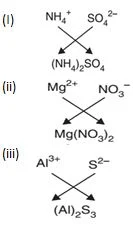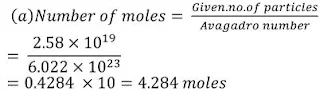#### Extra Questions for Class 9th: Ch 3 Atoms and Molecules (Science) Important Questions Answer Included

Very Short Answer Questions (VSAQs): 1 Mark

Q1.State the Law of Conservation of mass.
It states that mass can neither be created nor destroyed in a chemical reaction.

Q2. State the law of constant proportion.
Law of constant proportion states that in a chemical compound the elements are always present in a definite proportion by mass.

Q3. Explain what do you understand by valence electrons?
The electrons present in the outermost shell of an atom are called as valence electrons.

Q4. Name a non-metal that is tetra-atomic.
Phosphorus.

Q5. Give an example of:
(i) triatomic
(ii) polyatomic molecules of elements.
(i) Triatomic – Ozone O3
(ii) Polyatomic – Sulphur S8

Short Answer Questions-I (SAQs-I) : 2 Marks

Q 1. Write the chemical formula of:
(i) Sodium carbonate
(ii) Ammonium chlorideQ 2. (i) Explain what do you understand by Avogadro constant ?
(ii) Calculate the number of moles for 56 g of Ne. (Atomic mass Ne = 20 u)

(i) The exact number of atoms present in 12 gm of carbon-12 is called Avogadro constant.
(ii) No of moles = Given Mass/Molar Mass = m/M
= 56/20 = 2.8

Q4. (i) Write the chemical formula of a compound using zinc ion and phosphate ion.
(ii) Calculate the ratio by mass of atoms present in a molecule of carbon dioxide. (Given: C = 12, O = 16)

(i) Zn3(PO4)2(ii) The ratio by mass of constituting elements in carbon dioxide is:
CO2 = 12:(2 ×16) =12:32 = 3∶8

Short Answer Questions-II (SAQs-II) : 3 Marks

Q1. Show the formation of chemical formulae of following compounds using their ions:
(i) Ammonium sulphate
(ii) Magnesium nitrate
(iii) Aluminium sulphideQ 2. Derive the molecular formulae for the following compounds:
(i) Copper bromide
(ii) Ammonium carbonate
(iii) Aluminium oxide.

(i) (𝐶𝑢+2) (𝐵𝑟-1)➝ 𝐶𝑢𝐵𝑟2
(ii)( 𝑁𝐻4) + (𝐶𝑂3)-2 ➝ [𝑁𝐻4]2𝐶𝑂3
(iii) (𝐴𝑙)+3(𝑂)-2 ➝ 𝐴𝑙2𝑂3

Q3. Write the chemical symbols of the following elements: Gold, Copper, Potassium, Silver, Platinum and Iron.

Gold = Au, Copper = Cu, Potassium = K, Silver = Ag, Platinum = Pt, Iron = Fe.

Q4. (a) Answer the following questions:
(i) Name the scientist who discovered protons.
(ii) What is the charge and mass of a proton?
(iii) Where is proton located in an atom?
(b) An atom of an element has atomic mass 28 u and its atomic number is 14. How many neutrons does it have? Also name the element.

(a) (i) E. Goldstein
(ii) Mass of proton = 1.67×10-27 kgs
Charge on a proton = 1.67 × 10-19 coloumb
Also charge is + 1 and mass is 1 unit.
(iii) Protons are located in the nucleus.
(b) Number of neutrons = 28 – 14 = 14. Element is silicon.

Q5. What is meant by the term chemical formula? Write the chemical formula of calcium oxide. Calculate its formula unit mass. (Atomic mass of Ca = 40 u, O = 16 u)

CaO - Calcium oxide
Formula unit mass of CaO = Atomic mass of Ca + Atomic mass of O = 40 + 16 = 56 u

Long Answer Questions (LAQs) : 5 Marks

Q 1. (i) Name the international organization who approves names of elements.
(ii) Give an example with explanation to show that the law of conservation of mass applies to physical changes also.

(i) International Union of Pure and Applied Chemistry (IUPAC).

(ii) When ice melts into water it is a physical change. Take a piece of ice in a small flask, cork it and weigh it and denote it as Wice gm.
Heat the flask gently and ice (solid) slowly melts into water (liquid). Then, weigh the flask again as Wwater gm.
It is found that there is no change in the weight i.e., Wice = Wwater
This shows law of conservation of mass holds true for physical changes.

Q 2. (a) A sample of vitamin C is known to contain 2.58 ×1019 oxygen atoms. How many moles of oxygen atoms are present in the sample?
(b) Write one word for the following:
(i) In a balanced chemical equation, the sum of the masses of reactants and products remains unchanged.
(ii) A group of atoms carrying a fixed charge on them.
(c) Write chemical formulae of the following compounds:
(i) Sodium phosphate
(ii) Ammonium carbonate(b) (i) Law of conservation of mass
(ii) Ion
(c) (i) Na3PO4
(ii)( NH4)2CO3

Q3. (i) If 18 gm of pure water is electrolyzed, 2 gm of hydrogen and 16 gm of oxygen is obtained. Which law of chemical combination is illustrated by this statement?
(ii) State the law of constant proportion. Illustrate with the help of an example.
(iii)Which postulate of Dalton’s atomic theory is the result of law of conservation of mass?
(iv) Which point of Dalton’s atomic theory came from law of constant proportions ?